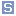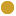## http://www.iec.ch/TC57/CIM#AsynchronousMachineEquivalentCircuit Class cim:AsynchronousMachineEquivalentCircuittypeClass [owl:Class]
commentThe electrical equations of all variations of the asynchronous model are based on the AsynchronousEquivalentCircuit diagram for the direct and quadrature axes, with two equivalent rotor windings in each axis. Equations for conversion between Equivalent Circuit and Time Constant Reactance forms: Xs = Xm + Xl X' = Xl + Xm * Xlr1 / (Xm + Xlr1) X'' = Xl + Xm * Xlr1* Xlr2 / (Xm * Xlr1 + Xm * Xlr2 + Xlr1 * Xlr2) T'o = (Xm + Xlr1) / (omega0 * Rr1) T''o = (Xm * Xlr1 + Xm * Xlr2 + Xlr1 * Xlr2) / (omega0 * Rr2 * (Xm + Xlr1) Same equations using CIM attributes from AsynchronousMachineTimeConstantReactance class on left of = sign and AsynchronousMachineEquivalentCircuit class on right (except as noted): xs = xm + RotatingMachineDynamics.statorLeakageReactance xp = RotatingMachineDynamics.statorLeakageReactance + xm * xlr1 / (xm + xlr1) xpp = RotatingMachineDynamics.statorLeakageReactance + xm * xlr1* xlr2 / (xm * xlr1 + xm * xlr2 + xlr1 * xlr2) tpo = (xm + xlr1) / (2*pi*nominal frequency * rr1) tppo = (xm * xlr1 + xm * xlr2 + xlr1 * xlr2) / (2*pi*nominal frequency * rr2 * (xm + xlr1).
subClassOfcim:AsynchronousMachineDynamics
equivalentClasscim:AsynchronousMachineEquivalentCircuit.xm max 1 cim:PUcim:AsynchronousMachineEquivalentCircuit.xlr2 max 1 cim:PUcim:AsynchronousMachineEquivalentCircuit.xlr1 max 1 cim:PUcim:AsynchronousMachineEquivalentCircuit.rr2 max 1 cim:PUcim:AsynchronousMachineEquivalentCircuit.rr1 max 1 cim:PU

Generated with TopBraid Composer by TopQuadrant, Inc.